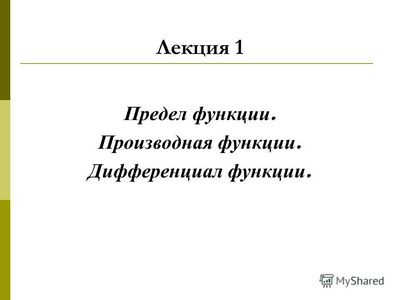# Высшая математика. Пределы, непрерывность, дифференцирование1. Limit of the sequence

Denition 1
A sequence can be thought of as a list of numbers written in a
denite order y1 , y2 , . . . yn, . . .
Denition 2
The number y1 is called the rst term, y2 is the second term,
and in general yn is the nth term.

A sequence can also be dened as a function whose domain is the
set of all positive integers. We usually write yn (or xn) instead of the
function notation for the (real) value at n.

N o t a t i o n 1
The sequence {y1, y2, . . .} is also denoted by {yn} or {yn}n=1 .
Denition 3

A sequence {xn} is called increasing if xn+1 > xn for all n

1,

that is x1 < x2 < . . . < xn < . . .. It is called decreasing if xn+1 < xn
for all n.
Denition 4

Non-increasing means xn+1

Denition 5

xn , non-decreasing : xn+1

xn.

A sequence xn is called monotonic if it is either non-decreasing
or non-increasing. It is called strictly monotonic if it is either decreasing
or increasing.

Denition 6
A sequence {xn} is called bounded if there is such a number M
that |xn | < M for all n 1.

E x a m p l e 1
xn= 1/n is decreasing. xn=

1 + (1)n
2

is bounded since |xn| <

2. The sequence xn=n is unbounded.
If we let xn be the digit in the nth decimal place of the number
e, the Euler number (to be dened later in the text), then xn is a
well-dened sequence but it doesn’t have a simple dening equation.

Denition 7
Fibonacci sequence xn is dened recursively by the conditions

x1 = 1, x2 = 1, xn = xn1 + xn2,

n

3.

Each term is the sum of the two preceding terms:

{1, 1, 2, 3, 5, 8, 13 . . .}

This sequence arose when the 13th-century Italian mathematician
Fibonacci solved a problem concerning the breeding of rabbits.
n

Consider the sequence

n + 1

. It appears that its terms are approaching

1 as n becomes large. In fact, the dierence
1 xn= 1n=

1

n + 1

n + 1

can be made as small as we like by taking n suciently large. Let
n

us indicate this by writing lim
n> n + 1

= 1.

In general, the notation lim
n>

= L means that the terms of the

sequence xn approach L as n becomes large.
Denition 8
A sequence {xn} has the limit L and we write lim
n>

xn= L or

xn > L as n > if we can make the terms xn as close to L as
we like by taking n suciently large. Ifnlim xnexists, we say the

>
sequence converges (or is convergent ). Otherwise, we say it diverges
(or is divergent ).

To make the above even more precise: L is the limit of xn, if for
an arbitrary positive there exists such N (which might depend on

) that |xn L| < as soon as n

N .

1.1.

Limit Laws for Convergent Sequences

If {an} and {bn} are convergent sequences and c is a constant,
then

lim (an+bn) = lim

an+ lim bn;

n>

n>

n>

lim (an bn) = lim

annlim

bn;

n>
lim
n>

n>
c • an=c lim
n>

an;

>

lim (anbn) = lim

an• lim

bn;

n>

lim

an

=

n>
lim
n>

an

,

n>

if

lim

bn= 0,

n> bn

lim bn
n>

n>

lim
n>

c = c.

Let us now consider a few situations which involve unbounded
sequences.

E x a m p l e 2

v

v

1) xn= 1/

n, yn=n,

xn • yn=

n > ;

2) xn=a/n, yn=n,
3) xn= 1/n2, yn=n,

xn • yn=a > a;
xn • yn= 1/n > 0;

4) xn= (1)n/n, yn=n,

xn • yn= (1)n, no limit.

In each case, we were in a 0 • indeterminate situation.
A few technicalities (when working with sequences).
n2 + 1

Let xn=

n2 1

. When trying to apply the respective limit law,

we get an indeterminate expression. A way to go is to divide both

5

the top and the bottom by n2:

1 + 1/n2
xn=1 1/n2=
1 1
where an = 1 + ,bn= 1 .

a
n
bn

,

n2

n2

Now, the limit law is applicable. We conclude that lim xn= 1.

n>
Later, when discussing the limit of a function, we will state a
theorem which deals with a limit of a rational function (that is, the

quotient of two polynomials).

v

v

v

Consider one other example. Let xn=

n(

n + 1

n). Again,

the limit law is inapplicable, we are in • 0 situation. A key word
here is “conjugate” (to try to get rid of one radical, at least). We

multiply xn by

1 =

vvn+ 1 + vvn
n + 1 + n

to get a new expression for the nth term:
vn
xn=vn+ 1 + vn,
still indeterminate expression.
We now do something similar to the previous example: we divide

both the numerator and the denominator by

v
n to get an expression

where we conclude easily about the existence of the limit:

1

1

xn=1 + 1/n + 1 >n> v1 + 0 + 1=12.
Denition 9
A sequence xn is bounded above if there is a number M such

that xn < M for all n

1.

It is bounded below if there is a number M such that xn > M for

all n

1.

6

(As we have dened earlier, if it is bounded above and below,
then xn is called a bounded sequence.)

Theorem

Комментарии к записи Высшая математика. Пределы, непрерывность, дифференцирование отключены

Рубрика: Разное

Обсуждение закрыто.